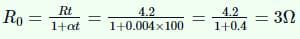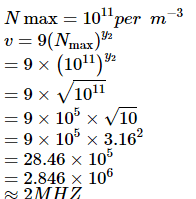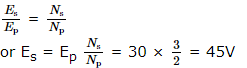JEE  >  VITEEE Physics Test - 2

# VITEEE Physics Test - 2

Test Description

## 35 Questions MCQ Test VITEEE: Subject Wise and Full Length MOCK Tests | VITEEE Physics Test - 2

VITEEE Physics Test - 2 for JEE 2023 is part of VITEEE: Subject Wise and Full Length MOCK Tests preparation. The VITEEE Physics Test - 2 questions and answers have been prepared according to the JEE exam syllabus.The VITEEE Physics Test - 2 MCQs are made for JEE 2023 Exam. Find important definitions, questions, notes, meanings, examples, exercises, MCQs and online tests for VITEEE Physics Test - 2 below.
Solutions of VITEEE Physics Test - 2 questions in English are available as part of our VITEEE: Subject Wise and Full Length MOCK Tests for JEE & VITEEE Physics Test - 2 solutions in Hindi for VITEEE: Subject Wise and Full Length MOCK Tests course. Download more important topics, notes, lectures and mock test series for JEE Exam by signing up for free. Attempt VITEEE Physics Test - 2 | 35 questions in 50 minutes | Mock test for JEE preparation | Free important questions MCQ to study VITEEE: Subject Wise and Full Length MOCK Tests for JEE Exam | Download free PDF with solutions
 1 Crore+ students have signed up on EduRev. Have you?
VITEEE Physics Test - 2 - Question 1

### The average power dissipation in pure inductance is

Detailed Solution for VITEEE Physics Test - 2 - Question 1

Solution :- power =1/T ∫v(t)i(t)dt

In sinusoidal wave, V and I differ by 90 °

Pa=ω2π∫Aωsin(ωt)cos(ωt)dt

where A is a constant depending on the value of the inductance L or capacitance C of the component.

P=Aω²/2π  [ ∫2π/sin(2ωt)dt]

period of sin(2ωt) is T′=π/ω

interval of integration of P is exactly two periods of sin(2ωt)

so finally :

P=Aω²/2π∫sin(2ωt)dt=0

VITEEE Physics Test - 2 - Question 2

### Moseley's law states that

Detailed Solution for VITEEE Physics Test - 2 - Question 2

The frequency of characteristic X-ray spectral line is directly proportional to the square of atomic number of target element.
⇒ v ∝ Z2 i . e . √ v ∝ Z
or √ v = a (z − b)
Here a is constant of proportionality b is screening constant.

VITEEE Physics Test - 2 - Question 3

### In a Wheatstone's bridge all the four arms have equal resistance R. If the resistance of the galvanometer arm is also R, the equivalent resistance of the combination as seen by the battery is

Detailed Solution for VITEEE Physics Test - 2 - Question 3

ln balance Wheatstone bridge, the galvanometer arm can be neglected so equivalent resistance = R.

VITEEE Physics Test - 2 - Question 4

Emission of electrons in the photoelectric effect is possible, if

VITEEE Physics Test - 2 - Question 5

A sheet of aluminium is inserted in the air gap between the parallel plate capacitor, without touching any the two plates of the capacitor. The capacitance the capacitor is:

VITEEE Physics Test - 2 - Question 6

When a current is passed through water acidified with a dilute sulphuric acid, the gases formed at the platinum electrodes are

VITEEE Physics Test - 2 - Question 7

The resistance of a coil at 100oC is 4.2 Ω. If temperature coefficient of resistance of the coil material is 0.004oC-1, then its resistance at 0oC is

Detailed Solution for VITEEE Physics Test - 2 - Question 7VITEEE Physics Test - 2 - Question 8

The internal resistance of a cell of e.m.f. 3 V is 0.1 Ω, If it is connected to a resistance of 2.9Ω then voltage across the cell will be

VITEEE Physics Test - 2 - Question 9

In short wave communication waves of which of the following frequencies will be reflected back by the ionospheric layer having electron density 1011 per m-3?

Detailed Solution for VITEEE Physics Test - 2 - Question 9VITEEE Physics Test - 2 - Question 10

A particle with a specific charge S is fired with a speed v towards a wall at a distance d, perpendicular to the wall. What minimum magnetic field must exist in this region for particle not hit the wall ?

Detailed Solution for VITEEE Physics Test - 2 - Question 10

The particle moves in a circular path with radius d if it is just to miss the wall
mv = Bqr
⇒ B = v ( q m ) d = v sd

VITEEE Physics Test - 2 - Question 11

When a current flows in a wire, there exists an electric field whose direction is

Detailed Solution for VITEEE Physics Test - 2 - Question 11

Solution :- The current always flows in the same direction as the electric field. The electrons flow in the opposite direction, because they are negatively charged.

VITEEE Physics Test - 2 - Question 12

A generator at a utility company produces 100 A of current at 4000 V. The voltage is stepped up to 240000 V by a transformer before it is sent on a high voltage transmission line. The current in transmission line is

VITEEE Physics Test - 2 - Question 13

A step-up transformer has transformation ratio of 3:2 What is the voltage in secondary, if voltage in primary is 30 V ?

Detailed Solution for VITEEE Physics Test - 2 - Question 13VITEEE Physics Test - 2 - Question 14

The coefficient of mutual inductance, when magnetic flux changes by 2 X 10-2 Wb and current changes by 0.01 A is

VITEEE Physics Test - 2 - Question 15

Which of the following waves have the maximum wavelength?

VITEEE Physics Test - 2 - Question 16

A point charge q is placed at a distance a/2 directly above the centre of a square of side a. The electric flux through the square assuming it to be one face of the cube is

VITEEE Physics Test - 2 - Question 17

A 2 μ F condenser is charged to 500V and then its plates are joined through of consistance. The heat produced in the resistance in joules is

VITEEE Physics Test - 2 - Question 18
Equal charges are given to two conducting spheres of different radii. The potential will
VITEEE Physics Test - 2 - Question 19

If a point charge q be rotated in a circle of radius r around a charge Q, the work done will be

VITEEE Physics Test - 2 - Question 20

The electric potential at a point (x, y) in the xy-plane is given by: V=-kxy. The electric field intensity at a distance r from the origin varies as

VITEEE Physics Test - 2 - Question 21

An electric dipole of dipole moment p is placed in uniform electric field of strength E. If θ is the angle between p and E, then potential energy of the dipole becomes maximum, when θ is

VITEEE Physics Test - 2 - Question 22
A straight wire of diameter 0.5 mm carrying a current of 1 A is replaced by another wire of 1 mm diameter carrying the same current. The magnetic field far away will be
VITEEE Physics Test - 2 - Question 23
The reason for the continuous emission of energy by the sun is
VITEEE Physics Test - 2 - Question 24

A radioactive element has a half life of 15 years. The fraction that will decay in 30 years is

VITEEE Physics Test - 2 - Question 25

The photoelectric threshold of a certain metal is 30 Å. If the radiation of 2000 Å is incident on the metal

VITEEE Physics Test - 2 - Question 26

A double convex lens made of air with both radii of curvature being 10 cm is placed in a medium of refractive index 1.5. The focal length and nature of lens is

VITEEE Physics Test - 2 - Question 27

In comparison to a half wave rectifier, the full wave rectifier gives lower

VITEEE Physics Test - 2 - Question 28

If a full wave rectifier circuit is operating from 50 Hz mains, then fundamental frequency in the ripple will be

VITEEE Physics Test - 2 - Question 29

The current gain for a transistor working as common-base amplifier is 0.96. If the emitter current is 7.2 mA, then the base current is

Detailed Solution for VITEEE Physics Test - 2 - Question 29

Solution :- Current gain of transistor is

α = ΔIC/ΔiE

Where ΔiC is change in collector current, and ΔiE is change in emitter current.

Given, α=0.96,ΔiE=7.2,

ΔiC=0.96×7.2=6.91mA

Also ΔiE=ΔiC+ΔiB

∴ ΔiB = ΔiE + ΔiC

= 7.2−6.91 = 0.29mA

VITEEE Physics Test - 2 - Question 30

To obtain electrons as majority charge carriers in a semiconductor, the impurity mixed is

VITEEE Physics Test - 2 - Question 31

The impurity atom that should be added to the germanium to make it an n-type semiconductor, is

VITEEE Physics Test - 2 - Question 32

In Young's double slit experiment, an interference pattern is obtained on a screen by a light of wavelength 6000 Å coming from the coherent sources S1 and S2. At certain point P on the screen third dark fringe is formed. Then the path difference S1 p − S2p in microns is

VITEEE Physics Test - 2 - Question 33

The phase difference between any two points on a wavefront is

VITEEE Physics Test - 2 - Question 34

A ray of light falls on a transparent glass slab of refractive index 1.62. If the reflected ray and the refracted ray are mutually perpendicular, the angle of incidence is

VITEEE Physics Test - 2 - Question 35

In the propagation of light waves, the angle between the direction of vibration and plane of polarisation is

## VITEEE: Subject Wise and Full Length MOCK Tests

1 videos|3 docs|73 tests
 Use Code STAYHOME200 and get INR 200 additional OFF Use Coupon Code
Information about VITEEE Physics Test - 2 Page
In this test you can find the Exam questions for VITEEE Physics Test - 2 solved & explained in the simplest way possible. Besides giving Questions and answers for VITEEE Physics Test - 2, EduRev gives you an ample number of Online tests for practice

## VITEEE: Subject Wise and Full Length MOCK Tests

1 videos|3 docs|73 tests